CUET  >  Solved Examples - Quadratic Equations

# Solved Examples - Quadratic Equations - General Test Preparation for CUET

Section - 1
Distribute the following expressions.
Ques 1: (x+ 2)(x- 3)
Ans:
(x + 2 )(x -3 )= x2- 3x + 2x - 6 = x2- x - 6

Ques 2: (2s+1)(s + 5)
Ans:
(2s+ 1)(s+5) = 2s2 + 10s + s + 5 = 2s2+ 11s + 5

Ques 3: (5 + a)(3 + a)
Ans:
(5 + a)(3 + a) = 15 + 5a + 3a + a2 = a2 + 8 a + 15

Ques 4: (3 - z)(z + 4)
Ans:
(3 - z)(z + 4) = 3z + 12 - z2 - 4z = -z2 - z + 12

Section - 2
Ques 5: x2 - 2x = 0
Ans:
x2 — 2x = 0
x(x — 2) = 0
x= 0
OR (x -2 ) = 0 -> x = 2

Ques 6: z2 = -5 z
Ans: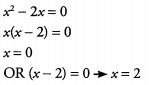Ques 7: y2 + 4y + 3 = 0
Ans: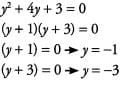Ques 8: y2- 11y + 30 = 0
Ans: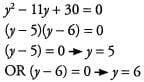Ques 9: y2 + 3 y = 0
Ans: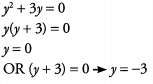Ques 10: y2 + 12y + 36 = 0
Ans: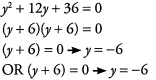Section - 3
Simplify the following expressions.
Ques 11: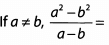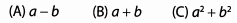Ans:
a + b1 The key to simplifying this expression is to recognize the special product: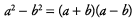After replacing the original numerator with (a + b)(a - b), we can cancel the (a - b) in the numerator with the (a - b) in the denominator: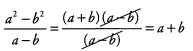Ques 12: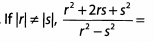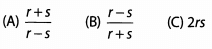Ans: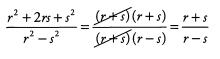Ques 13: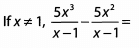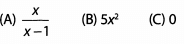Ans: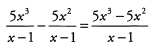Because we have a common factor in both terms of the numerator, we can divide that factor out in order to simplify further. This is often a useful move when we are asked to add or subtract exponents with the same base: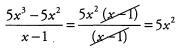Ques 14: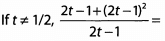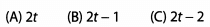Ans: It is tempting to expand the quadratic term in the numerator, but we should try to simplify first. Notice that none of the answer choices are fractions. Therefore, we need to look for a way to cancel (2t — 1) from the denominator. To make this task easier, enclose every (2t — 1) term in parentheses and then simplify. We can factor (2t - 1) out of the numerator: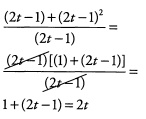Alternatively, we can introduce a new variable x defined as x = 2t — 1. In general, it is not a good idea to introduce more variables than strictly necessary, but in this case the new variable can make it easier to see how the math works: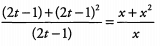We can then simplify the expression in terms of x: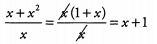And we then finish by replacing x with 2t - 1
x + 1 = (2t — 1) + 1 = 2t

Section - 4
Simplify the following expressions.
Ques 15: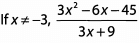(A) 5 - x (B) x - 5 (C) x + 5
Ans:
We can make this problem a lot simpler if we begin by factoring 3 out of both the nu-

merator and the denominator: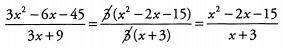The answers are not fractions, so well have to get rid of the denominator. Factor the numerator: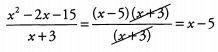Ques 16: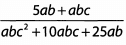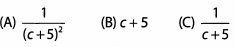Ans:
This might seem nearly impossible to factor down until we take out the common term: ab. Then, we are left with one of our familiar special products in the denominator: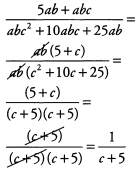Ques 17: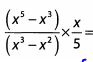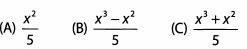Ans:
There's no obvious way to proceed through this question. The best bet is to try to

simplify before multiplying. Notice that x3 can be factored out of the numerator and x2 can be factored out of the denominator: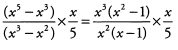Notice now that we can cancel x2, from the fraction. Also, we have (x2 - 1) in the numerator, which we can factor: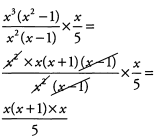We don’t have a match with any of the answer choices yet. None of the numerators in the answer choices have parenthetical expressions. We should multiply the numerator: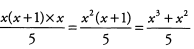The document Solved Examples - Quadratic Equations | General Test Preparation for CUET is a part of the CUET Course General Test Preparation for CUET.
All you need of CUET at this link: CUET

## General Test Preparation for CUET

176 videos|330 docs|400 tests

## FAQs on Solved Examples - Quadratic Equations - General Test Preparation for CUET

 1. What is a quadratic equation?Ans. A quadratic equation is a second-degree polynomial equation in a single variable, where the highest power of the variable is 2. It can be written in the form ax^2 + bx + c = 0, where a, b, and c are constants, and a ≠ 0.
 2. How do you solve a quadratic equation?Ans. To solve a quadratic equation, you can use factoring, completing the square, or the quadratic formula. Factoring involves finding the factors of the quadratic equation and setting each factor equal to zero. Completing the square involves manipulating the equation to create a perfect square trinomial. The quadratic formula is a general formula that gives the solutions to any quadratic equation.
 3. Why are quadratic equations important?Ans. Quadratic equations are important in various fields such as physics, engineering, finance, and computer science. They help in modeling real-life situations involving parabolic curves, projectile motion, optimization problems, and more. Quadratic equations also form the basis for understanding higher-level mathematical concepts.
 4. What is the discriminant of a quadratic equation?Ans. The discriminant of a quadratic equation is a value that helps determine the nature of the solutions. It is calculated as Δ = b^2 - 4ac, where a, b, and c are the coefficients of the quadratic equation. If the discriminant is greater than zero, the equation has two distinct real solutions. If it is equal to zero, the equation has only one real solution. If the discriminant is less than zero, the equation has no real solutions.
 5. Can a quadratic equation have no solutions?Ans. Yes, a quadratic equation can have no real solutions if the discriminant is negative. In such cases, the solutions are complex numbers, involving the imaginary unit "i." However, it is important to note that every quadratic equation will always have two solutions, whether they are real or complex.

## General Test Preparation for CUET

176 videos|330 docs|400 testsExplore Courses for CUET examSignup to see your scores go up within 7 days! Learn & Practice with 1000+ FREE Notes, Videos & Tests.
10M+ students study on EduRev
Track your progress, build streaks, highlight & save important lessons and more!
Related Searches

,

,

,

,

,

,

,

,

,

,

,

,

,

,

,

,

,

,

,

,

,

;